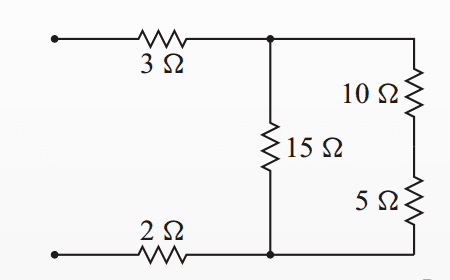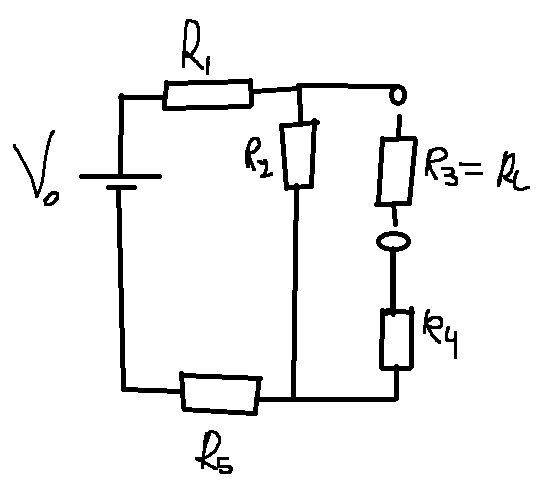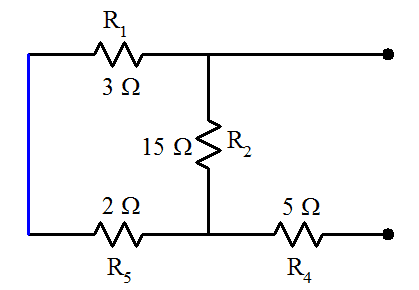# Thevenin's Theorem Problem

• Potatochip911

## Homework Statement

Determine the voltage across ##R_3## in the following figure assuming an input voltage ##v_0## of 10V is applied across the open terminals.Let ##R_1=3\Omega##, ##R_2=15\Omega##, ##R_3=10\Omega##, ##R_4=5\Omega## and ##R_5=2\Omega## and

## The Attempt at a Solution

So I'm trying to use Thevenin's theorem to solve this, I already solved it using basic current/voltage rules and I obtained ##V_{R3}=4V##, if I set ##R_3## as the load resistor I obtain the following circuit:Now I can't see how to find ##R_{th}## by shorting the voltage source and then combining the resistors, I believe I can just remove ##R_4## in that scenario but then I have ##R_1##, ##R_2## and ##R_3## connected together and I'm not sure which of these two are in parallel (if someone could explain how to go about solving it this way that would be great). Because of this I decided to find ##R_{th}## using ##V_{th}## and the open circuit current, if I short ##R_3## then we have ##R_2##//##R_4## so by a voltage divider we obtain ##I_{sc}=\frac{v_0}{R_1+\frac{R_2R_4}{R_2+R_4}+R_5}##. Now to find the voltage ##V_{th}## we need the voltage across ##R_3##, I would've thought that it would just be ##V_{th}=\frac{V_0}{R_1+R_2+R_5}R_2##. However, this doesn't lead to the correct answer after calculating ##R_{th}## using ##V_{th}=I_{sc}R_{th}## and then building the thevenin equivalent circuit.

You can't ignore R4 as it contributes to the Thevenin resistance. After all, it is in series with one of the output terminals.

Removing the load resistor R3 and shorting the voltage supply leaves you with:What can you say about the relationship between R1 and R5?

•Potatochip911
You can't ignore R4 as it contributes to the Thevenin resistance. After all, it is in series with one of the output terminals.

Removing the load resistor R3 and shorting the voltage supply leaves you with:
View attachment 98762

What can you say about the relationship between R1 and R5?
Yea I thought I understood thevenin's theorem but I clearly didn't. For anyone who stumbles upon this thread and is curious I found this video very helpful. I essentially wasn't looking at stuff from the perspective of where the load is removed before, after taking this into consideration we find that ##R_{th}=(R_1+R_5)//R_2+R_4## which can be be figured out quite easily if you consider a voltage source in place of the open circuit in the image gneill made and consider where the current flows. Now for ##V_{th}## the we need to consider the circuit from the point of view of the input voltage with the open circuit where the resistor was, therefore it becomes a simple series circuit and since we want voltage across ##R_2## we find ##V_{th}=\frac{v_0}{R_1+R_2+R_5}R_2##. building the thevenin circuit using these values and calculating voltage across ##R_3## will give the correct answer of 4V.